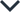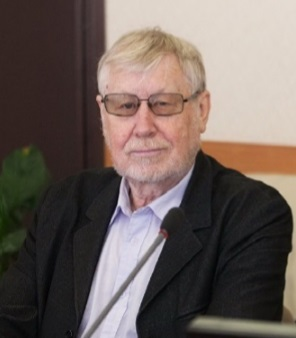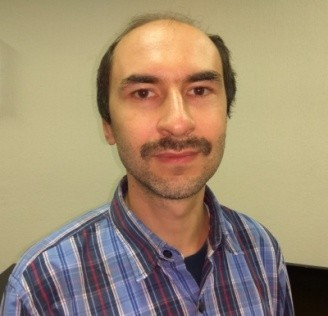Ph.D. Supervisors in Mathematics and Artificial Intelligence

Kudryashov Nikolay AlekseyevichKudryashov Nikolay Alekseyevich — Doctor of Physical and Mathematical Sciences, professor. Head of Department of Applied Mathematics at the National Research Nuclear University MEPhI.

Honored Scientist of the Russian Federation, USSR State Prize winner, Russian Federation Government Prize winner (in Education).

University: National Research Nuclear University MEPhI (Moscow Engineering Physics Institute).

English proficiency: fluent.

Programme: Applied Mathematics and Information Science (Nonlinear differential equations).

Direction: 01.06.01 Mathematics and Mechanics.

# List of Research Projects (participation/management):

Russian Science Foundation, Russian Foundation for Basic Research

• Russian Science Foundation, 14-11-00258, “Nonlinear mathematical models and methods for their investigation” grant for fundamental research and exploratory research by individual research groups, 2014-2016;
• Russian Science Foundation, 18-11-00209, “Development of methods for investigation of nonlinear mathematical models” grant for fundamental research and exploratory research by individual research groups, 2018- 2020;
• Russian Foundation for Basic Research, 18-29-10025, “Analytical, asymptotic and numerical solutions of higher analogs of Painlevé equations” grant, 2019-2021.

# List of possible research topics:

• Painlevé methods for finding exact solutions to nonlinear mathematical models in Optics;
• Analytical characteristics of higher analogs of Painlevé equations.

Field of Study: Nonlinear mathematical models and methods for their research.

Research interests: Nonlinear mathematical models, nonlinear differential equations, Painlevé methods, Painlevé equations and Painlevé-type equations, nonlinear waves, dynamic systems, nonlinear optics methods, analysis of dynamic

processes and chaos, analytical and numerical methods of intergrability and finding solutions.

Specified requirements for a candidate: mathematical education and publication activity.

# Number of publications in Web of Science and Scopus:

Number of publications in Web of Science -110

Number of publications in SCOPUS -108

My publications:

• Kudryashov, N.A. Solitary wave solutions of hierarchy with non-local nonlinearity. (2020) Applied Mathematics Letters, 103, art. no. 106155. (IF- 3.848);
• Kudryashov, N.A. Traveling wave reduction of the modified KdV hierarchy: The Lax pair and the first integrals. (2019) Communications in Nonlinear Science and Numerical Simulation, 73, pp. 472-480. (IF -4.115);
• Kudryashov, N.A. Highly dispersive solitary wave solutions of perturbed nonlinear Schrödinger equations. (2020) Applied Mathematics and Computation, 371, art. no. 124972. (IF – 3.472));
• Kudryashov, N.A. Nonlinear differential equations associated with the first Painlevé hierarchy. (2019) Applied Mathematics Letters, 90, pp. 223-228. (IF - 3.848);
• Kudryashov, N.A. Exact solutions of the equation for surface waves in a convecting fluid. (2019) Applied Mathematics and Computation, 344-345, pp. 97-106. (IF - 3.472).

# Significant results of intellectual activity:

The obtainment of the first integrals and the general solution of the generalized Gerdjikov-Ivanov equation (N. A. Kudryashov, Traveling wave solutions of the generalized Gerdjikov-Ivanov equation, Optik, 219 (2020) 165193);

The obtainment of the first integrals and the general solutions in variables of the traveling waves of the complex Ginzburg-Landau equation (N. A. Kudryashov, First integrals and general solutions of the complex Ginzburg-landau equation, Applied Mathematics and Computation, 386 (2020) 125407);

The obtainment of the periodic and solitary waves of the generalized Biswas- Arshed equation applied in nonlinear optics (Kudryashov, N. A. Solitary wave solutions of the generalized Biswas-Arshed equation. Optik (Stuttg). 219, (2020)).

The obtainment of the first integrals and the general solutions of the Biswas- Milovic equation applied in nonlinear optics (Kudryashov, N. A. First integrals and general solutions of the Biswas-Milovic equation. Optik (Stuttg). 210, (2020)).

The obtainment of the periodic and solitary waves of the equation in the Bragg gratings with dispersive scattering (Kudryashov, N. A. Periodic and solitary waves in optical fiber Bragg gratings with dispersive reflectivity. Chinese J. Phys. 66, 401–405 (2020)).

The finding of the solitary waves of a hierarchy of equations with non-local nonlinearity (Kudryashov, N. A. Solitary wave solutions of hierarchy with non- local nonlinearity. Appl. Math. Lett. 103, (2020)).

The construction of the Lax pairs and the obtainment of the special solutions of equations associated with self-similar reductions of the Sawada-Kotera and Kupershmidt equations (Kudryashov, N. A. Lax Pairs and Special Polynomials Associated with Self-similar Reductions of Sawada — Kotera and Kupershmidt Equations. Regul. Chaotic Dyn. 25, 59–77 (2020)).

The proposal of a new version of the method for constructing solitary waves of nonlinear partial differential equations, the efficiency of which is especially useful in finding optical solitons (Kudryashov, N. A. Method for finding highly  dispersive optical solitons of nonlinear differential equations. Optik (Stuttg). 206, (2020)).

The proposal of the nonlinear second-order and fourth-order equations with envelope solitons for description of solitary waves in optical fiber (Kudryashov, N.

A. Construction of nonlinear differential equations for description of propagation pulses in optical fiber. Optik (Stuttg). 192, 138–144 (2019); Kudryashov, N. A. A generalized model for description of propagation pulses in optical fiber. Optik (Stuttg). 189, 42–52 (2019); Kudryashov, N. A. Mathematical model of propagation pulse in optical fiber with power nonlinearities. Optik (Stuttg). 212, (2020)).

The finding of the first integrals and the general solution of the Fokas-Lenells equation to describe waves in nonlinear optics (Kudryashov, N. A. First integrals and general solution of the Fokas–Lenells equation. Optik (Stuttg). 195, 163135 (2019)).

The construction of the general solution of the traveling wave reduction for the perturbed Chen-Lee-Liu equation for describing optical solitons (Kudryashov, N.

A. General solution of the traveling wave reduction for the perturbed Chen-Lee- Liu equation. Optik (Stuttg). 186, 339–349 (2019)).

The obtainment of the general solution of the equation of the Kundu–Mukherjee– Naskar model that is invariant under transformations of the shift group

(Kudryashov, N. A. General solution of traveling wave reduction for the Kundu– Mukherjee–Naskar model. Optik (Stuttg). 186, 22–27 (2019)).

The obtainment of the first integrals and general solution of the generalized Schrödinger equation with anti-cubic nonlinearity (Kudryashov, N. A. First integrals and general solution of the traveling wave reduction for Schrödinger equation with anti-cubic nonlinearity. Optik (Stuttg). 185, 665–671 (2019)).

The obtainment of the first integrals and the general solution of the Triki-Biswas equation for describing optical solitons (Kudryashov, N. A. First integrals and solutions of the traveling wave reduction for the Triki–Biswas equation. Optik (Stuttg). 185, 275–281 (2019)).

The implementation of the Painlevé approach for constructing solitary wave solutions of nonintegrable differential equations (Kudryashov, N. A. The Painlevé approach for finding solitary wave solutions of nonlinear nonintegrable differential equations. Optik (Stuttg). 183, 642–649 (2019)).

Trofimov Aleksandr GennadievichTrofimov Aleksandr Gennadievich — Doctor of Science, senior lecturer of the Department of Cybernetics at the Institute of Cyber Intelligent Systems of the National Research Nuclear University MEPhI.

Research advisor of the Laboratory of Neural networks technologies at the Institute of Cyber Intelligent Systems of the National Research Nuclear University MEPhI, member of the editorial board of the Journal Neurocomputers, member of program committee and organizing committee of the international conference "NEUROINFORMATICS".

University: National Research Nuclear University MEPhI (Moscow Engineering Physics Institute).

English proficiency: fluent.

Programme: Applied Mathematics and Information Science, Software Engineering.

Directions:

09.06.01 Computer Science and Computer Facilities (100)

# List of Research Projects (participation/management):

• Russian Foundation for Basic Research, 15-29-01344, 2015-2017, participation.

Analysis of wave-like processes in the human brain.

• Russian Science Foundation, 14-28-00234, 2015–2017, participation.

In search of the "I": Interdisciplinary research on the initiation of voluntary action.

# List of possible research topics:

1. Development of an intelligence system for image geotagging.
2. Vulnerability study of existing types of graphic captcha as a cyber security elements. Development of a computer-aided system for captcha recognition and generation of hack-resistant captcha types.
3. Research of the success of start-ups based on open source text analysis, including social networks.
4. Development of a predictive analytics system for early detection of breakdowns at power plants.
5. Development of methods for detecting adversarial attacks on ML models and methods of protection against them.

Field of Study: Machine learning and neural networks.

# Research interests:

1. Image processing and computer vision.
2. Analysis of natural language texts.
3. Predictive analytics.
4. Machine learning models security.

Key features of the programme: Opportunity to work with MEPhI graphics accelerators of the Tensor computing module (1280 GPU tensor cores, 10240 CUDA cores).

# Specified requirements for a candidate:

• Knowledge of mathematical analysis, linear algebra, probability theory and mathematical statistics
• Python and MATLAB skills;
• Knowledge of Python scientific programming libraries, including scikit-learn.

# Number of publications in Web of Science and Scopus (9 publications):

• Trofimov A.G., Bogatyreva A.A. A method of choosing a pre-trained convolutional neural network for transfer learning in image classification problems // Studies in Computational Intelligence, 2020, Vol. 856 pp. 263-270.
• Trofimov A.G., Kuznetsova K.E., Korshikova A.A. Abnormal Operation Detection in Heat Power Plant Using Ensemble of Binary Classifiers //  Advances in Neural Computation, Machine Learning, and Cognitive Research, 2019, V.799, Pp.227-233.
• Trofimov A.G., Shishkin S.L., Kozyrskiy B.L., Velichkovsky B.M. A Greedy Feature Selection Algorithm for Brain-Computer Interface Classification Committees // Procedia Computer Science, 2018, V.123, Pp. 488-493.
• Trofimov A.G., Velichkovsky B.M., Shishkin S.L. An Approach to Use Convolutional Neural Network Features in Eye-Brain-Computer-Interface // Studies in Computational Intelligence, 2018, V.736, Pp.132-137.
• Shishkin, Y. Nuzhdin, E. Svirin, A. Trofimov, A. Fedorova, B. Kozyrskiy, B. Velichkovsky. EEG Negativity in Fixations Used for Gaze-Based Control: Toward Converting Intentions into Actions with an Eye-Brain-Computer Interface // Frontiers in Neuroscience. 2016, V.10, P. 1-20.